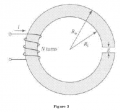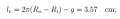# Need help with magnetic circuit (mean path length)

Thread Starter

#### 김찬우 1

Joined Oct 8, 2018
11(sorry for my bad english)

ri=3.4cm, ro=40.cm

and the question is calculating the mean core length, and the answer isbut i cant understand how this calculation came out.

i think it should be 2*pi*(r0+ri)/2=pi(r0+ri),, i need explanations for this problem.

thanks

Last edited:

#### wayneh

Joined Sep 9, 2010
17,152
Deriving the answer requires a good application of some calculus skills. Otherwise you have to accept the solution, that the mean circumference depends on the mean radius, which is at least easy to remember.

Oops I missed that the given answer is crap.

Last edited:
Thread Starter

#### 김찬우 1

Joined Oct 8, 2018
11
you meanthis answer is wrong?

#### ebp

Joined Feb 8, 2018
2,332
EDIT - made a mess of this the first time; should be OK now

Your equation is correct. If g is zero
le = π(Ro+Ri)

Here's a datasheet for a powdered iron core I've used many times:
micrometalsarnoldpowdercores.com/pdf/T106-52-DataSheet.pdf

Note that the specifications for dimensions are the inner and outer diameters in millimetres and the path length is specified in centimetres. Your equation gives the corrrect path length.

Last edited:
Thread Starter

#### 김찬우 1

Joined Oct 8, 2018
11
thanks for the replying. it helped me a lot.

edit - can i ask you one more?

the question says that the iron is of infinite permeability and neglect the effects of magnetic leakage and fringing.

Do this assumption affects the calculation of mean core length?

#### wayneh

Joined Sep 9, 2010
17,152
thanks for the replying. it helped me a lot.

edit - can i ask you one more?

the question says that the iron is of infinite permeability and neglect the effects of magnetic leakage and fringing.

Do this assumption affects the calculation of mean core length?
Nope. If the permeability was not uniform with radius, that could have an effect.

#### MrAl

Joined Jun 17, 2014
8,251
View attachment 161191

(sorry for my bad english)

ri=3.4cm, ro=40.cm

and the question is calculating the mean core length, and the answer is
View attachment 161193

but i cant understand how this calculation came out.

i think it should be 2*pi*(r0+ri)/2=pi(r0+ri),, i need explanations for this problem.

thanks
Hello,

Hard to verify exactly if you dont give the value of 'g', but they may be implying that g=0.2 perhaps. We also have to assume that 40 is really 4.0 in that question.

With g=0 yes the mean mag path length is:
pi*(Ro+Ri)

To determine if the mean mag path length is:
pi*(Ro+Ri)-g

where the two core face surfaces are perfectly parallel we'd have to dig a little deeper, but for very small 'g' i think it should be a good enough estimate.

The fact that the core permeability is infinite may change things too though because then the gap doesnt have any effect. That is if this is a little bit of a tricky question where it really is infinite and not just "large".

#### wayneh

Joined Sep 9, 2010
17,152
The fact that the core permeability is infinite may change things too though because then the gap doesnt have any effect.
I don't think you mean that. The gap is still a critical piece and it's size matters.

#### wayneh

Joined Sep 9, 2010
17,152
you meanthis answer is wrong?
It might, by coincidence, produce the right result in this example. But the formula is incorrect. If anyone challenges you on that, tell them to imagine a toroid with Ro of 100 and Ri of 99. Do they really believe the mean core length is just 2π?

#### MrAl

Joined Jun 17, 2014
8,251
With reference to the gap size being insignificant if the permeability is infinite...
I don't think you mean that. The gap is still a critical piece and it's size matters.
Hi,

Well go through the calculation of inductance and see what you end up with.
I may have inverted the logic here, where instead the air gap now has total control over the average permeability.
From the point of view of reluctance it looks like the air gap takes control over the total reluctance completely.
So instead of zero control it has total controlI'll look at this too in more detail later.

Similar threads